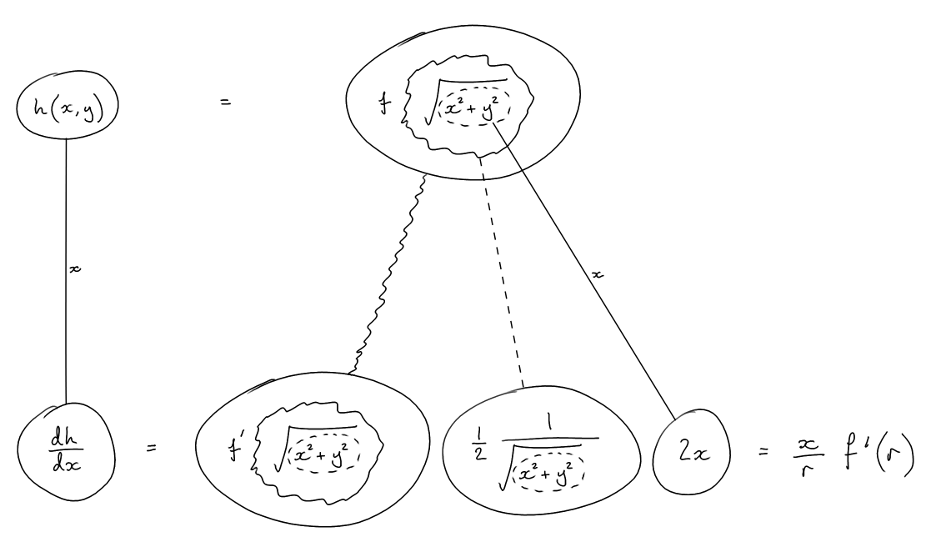# Thread: Directional derivatives and partial derivatives

1. ## Directional derivatives and partial derivatives

Suppose f: R -> R is differentiable and let h(x,y) = f(√(x^2 + y^2)) for x ≠ 0. Letting r = √(x^2 + y^2), show that:

x(dh/dx) + y(dh/dy) = rf'(r).

I have begun by showing that rf'(r) = sqrt(x^2 + y^2) * limt->0 (f(r+t) - f(r))/t

and written out the definition form of the directional derivatives. I cant seem to find a way to equate both sides of the equation. Can anyone help?

2. ## Re: Directional derivatives and partial derivatives

Hey tlawrence.

What did you calculate dh/dx and dh/dy in terms of the function f^n(r)? (Note: f^n(r) is the nth derivative of the function f with argument r)?

3. ## Re: Directional derivatives and partial derivatives

Also/anyway, f(r) and r(x,y) are differentiable so you can apply the chain rule...... where (key in spoiler) ...

Spoiler:... is the chain rule. Straight continuous lines differentiate downwards (integrate up) with respect to the main variable (in this case x or y), and the straight dashed line similarly but with respect to the dashed balloon expression (the inner function of the composite which is subject to the chain rule).

Similarly with dh/dy. Then substitute into the left-hand side of the show-sentence.

_________________________________________

Don't integrate - balloontegrate!

Balloon Calculus; standard integrals, derivatives and methods

Balloon Calculus Drawing with LaTeX and Asymptote!

#### Search Tags

derivatives, directional, partial, shifrin, vector calculus